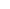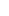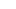# Forex Smart Trade Results, Friday, June 9, 2023 – \$9,867###### Forex Smart Trade Results, Thursday, June 8, 2023 – \$0
June 17, 2023June 21, 2023

# The MACD Line.

The Signal Line is the moving average of the MACD Line.

When looking at the indicator, we consider the Signal Line the “slower” moving average.

The slower-moving average plots the average of the previous MACD Line.

Once again, from our example above, this would be a 9-period moving average.

Most charts use a 9-period exponential moving average (EMA) by default.

This means that we are taking the average of the last 9 periods of the “faster” Moving Average Convergence Divergence Line and plotting it as our “slower” moving average.

The purpose of the Signal Line is to smooth out the sensitivity of the MACD Line.

The Histogram simply plots the difference between the MACD Line and Signal Line.

It is a graphical representation of the distance between the two lines.

It may sometimes give you an early sign that a crossover is about to happen.

If you look at our original chart, you can see that, as the two moving averages (Moving Average Convergence Divergence Line and Signal Line) separate, the histogram gets bigger.

## Divergence

This is called a MACD divergence because the faster-moving average (Moving Average Convergence Divergence Line) is “diverging” or moving away from the slower-moving average (Signal Line).

As the moving averages get closer to each other, the histogram gets smaller.

This is called convergence because the faster-moving average (MACD Line) is “converging” or getting closer to the slower-moving average (Signal Line).

And that, my friend, is how you get the name, Moving Average Convergence Divergence!